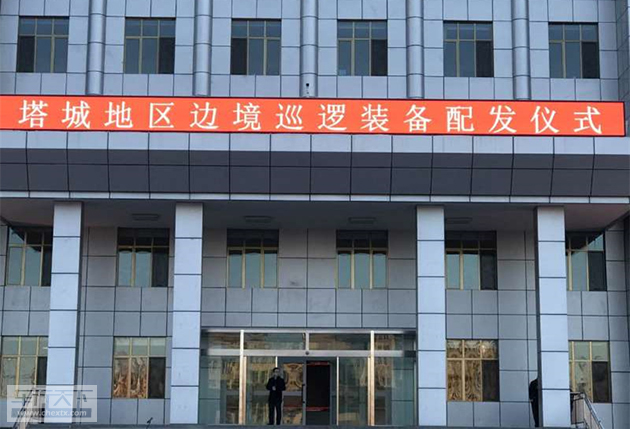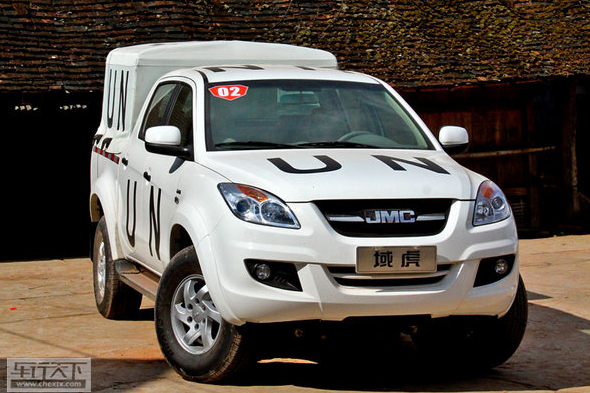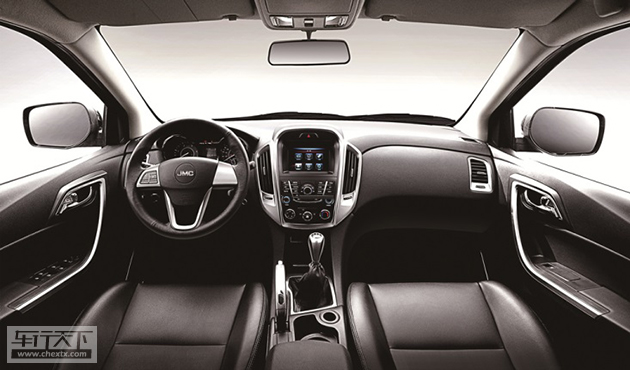## 国门新卫士 150台江铃域虎驻守新疆边境线

2016年12月21日 14:41 来源：www.chextx.com 超过：次关注

12月的新疆塔城地区，一片银装素裹，在蓝天白雪的映衬下，显得愈发静谧安宁。然而，这里却是中国的边防重地，塔城辖区内有5个县(市)与哈萨克斯坦共和国接壤，边境线长达546公里。在这个大雪时节，塔城边防队迎来了一群热血澎湃的“中国虎”——全疆150台江铃域虎边防用车首批18台在这里隆重发车!整齐排列在广场上的域虎显得庄严肃穆，爱国之情早已印刻在钢筋铁骨中，报国之路从这里启程!其实在“出生”之时，域虎的家国情怀就已然融汇在品牌文化里。

域虎品牌诞生于自主皮卡突破求变之年。2012年， 域虎作为国内第一款引进美式宽体皮卡理念的自主高端皮卡震撼上市，打破了外资对国内中高端皮卡市场的垄断，为自主品牌皮卡树立起了一道国门。“勇者征途——江铃域虎国门之旅”由此应运而生。五年来，域虎的脚步遍布一座座边陲小城，探寻国门文化，致敬国门卫士，巡礼国门昌荣。从北疆国门黑河、中国北大门二连浩特、东方雨林西双版纳、东亚之窗满洲里，一直到今年的最美国门瑞丽，域虎以卓越的产品力和切实的行动力，在国门呐喊中国皮卡的未来，用车轮描绘自主品牌的昌荣。正如“让世界向你看齐”的slogan，域虎驰骋国门之境，以纵横疆场的果敢精神、纯正的越野行车体验，向国人展示自主皮卡的另一番风貌，也向世界彰显中国品牌的魅力。爱国靠信念，报国靠实力!江铃域虎的家国情怀，不止是一场巡礼国门的活动，更是经过了铁血军营的锤炼和磨砺。

早在2012年上市之初，域虎就凭借高品质，成功入围中国人民解放军后勤用车。之后又经过层层选拔入选联合国维和部队车辆，在2014年7月至2015年3月期间，与中国维和部队在西非利比里亚执行了8个多月的维和任务。在去年的反法西斯战争胜利70周年大阅兵，以及今年的长征7号火箭发射现场，域虎都作为后勤保障车辆出镜。前不久，域虎又踏上了友好交流大使的征程，在中国军援科摩罗的项目中，成为中方的主要军援装备，交付科摩罗军队成为制式装备。如今，150虎将又相继奔赴新疆维吾尔自治区的各个边防地区，为祖国的边防事业做贡献!能在各种军工领域获得认可，江铃域虎这位军旅硬汉的实力，必当非同小觑。

域虎搭载的源自福特的PUMA2.4L TDCI柴油发动机，低转高扭优势明显，爆发力惊人。非承载式车身配以225mm的最小离地间隙，同级别车型最优。配备螺旋弹簧的双横臂式前悬，使车辆在高速行驶时抗扭曲能力更强。由英国莲花公司精心调校的底盘和悬挂系统，使得整车配适性一流。在安全性能和配置上，域虎也下足了功夫。ABS+EBD系统、车载蓝牙、导航、多功能方向盘、双安全气囊等设备，让这款霸气的皮卡在安全配置方面趋于全面，确保行车路上的畅行无忧。动力强、适应佳、多用途、高安全——域虎用自己的铁血基因，践行着爱国、报国的情怀。在这位军营“老兵”身上，我们看到了不断提升、愈战愈勇的精神。愿更多自主品牌能雄起，为中华民族的伟大复兴贡献力量!

#### 相关文章

﻿
• 快速找车
• 选择品牌
• 选择品牌
• A  奥迪
• A  阿斯顿·马丁
• A  阿尔法·罗密欧
• B  宝沃
• B  布加迪
• B  巴博斯
• B  保时捷
• B  宾利
• B  奔驰
• B  宝马
• B  本田
• B  别克
• B  标致
• B  比亚迪
• B  宝骏
• B  北汽制造
• B  北汽新能源
• B  北汽幻速
• B  北汽威旺
• B  北京汽车
• B  奔腾
• B  北汽绅宝
• B  北汽昌河
• C  长安欧尚
• C  长安
• C  长安凯程
• C  长城
• D  大众
• D  道奇
• D  DS
• D  东南
• D  东风风神
• D  东风风行
• D  东风小康
• D  东风风度
• D  东风
• F  福特
• F  丰田
• F  菲亚特
• F  法拉利
• F  福田
• F  福迪
• F  福汽启腾
• G  观致
• G  广汽传祺
• G  广汽吉奥
• G  GMC
• H  红旗
• H  汉腾汽车
• H  哈弗
• H  哈飞
• H  海格
• H  海马
• H  华颂
• H  黄海
• H  华泰
• H  恒天
• J  几何汽车
• J  捷达
• J  吉利汽车
• J  捷豹
• J  Jeep
• J  江淮
• J  江铃
• J  金杯
• J  九龙
• J  金旅
• K  凯翼
• K  凯迪拉克
• K  克莱斯勒
• K  科尼塞克
• K  卡威
• K  开瑞
• L  路虎
• L  林肯
• L  劳斯莱斯
• L  兰博基尼
• L  雷克萨斯
• L  铃木
• L  领克
• L  雷诺
• L  理念
• L  力帆
• L  莲花汽车
• L  猎豹
• L  路特斯
• L  陆风
• M  马自达
• M  MG
• M  MINI
• M  玛莎拉蒂
• M  摩根
• M  迈凯轮
• N  纳智捷
• O  欧拉
• O  欧宝
• O  讴歌
• O  欧朗
• Q  奇瑞
• Q  起亚
• Q  启辰
• R  日产
• R  荣威
• R  瑞麒
• S  SERES赛力斯
• S  三菱
• S  斯威汽车
• S  萨博
• S  smart
• S  斯柯达
• S  斯巴鲁
• S  思铭
• S  双龙
• S  上汽大通
• S  双环
• T  特斯拉
• T  腾势
• W  蔚来
• W  沃尔沃
• W  WEY
• W  五菱汽车
• W  五十铃
• W  威兹曼
• W  威麟
• X  现代
• X  雪佛兰
• X  星途
• X  雪铁龙
• X  小鹏汽车
• X  西雅特
• Y  一汽
• Y  英菲尼迪
• Y  英致
• Y  依维柯
• Y  野马汽车
• Y  永源
• Z  众泰
• Z  中华
• Z  中兴
• Z  知豆
• 选择车系
• 选择车系
• 车型对比
• 选择品牌
• 选择品牌
• A  奥迪
• A  阿斯顿·马丁
• A  阿尔法·罗密欧
• B  宝沃
• B  布加迪
• B  巴博斯
• B  保时捷
• B  宾利
• B  奔驰
• B  宝马
• B  本田
• B  别克
• B  标致
• B  比亚迪
• B  宝骏
• B  北汽制造
• B  北汽新能源
• B  北汽幻速
• B  北汽威旺
• B  北京汽车
• B  奔腾
• B  北汽绅宝
• B  北汽昌河
• C  长安欧尚
• C  长安
• C  长安凯程
• C  长城
• D  大众
• D  道奇
• D  DS
• D  东南
• D  东风风神
• D  东风风行
• D  东风小康
• D  东风风度
• D  东风
• F  福特
• F  丰田
• F  菲亚特
• F  法拉利
• F  福田
• F  福迪
• F  福汽启腾
• G  观致
• G  广汽传祺
• G  广汽吉奥
• G  GMC
• H  红旗
• H  汉腾汽车
• H  哈弗
• H  哈飞
• H  海格
• H  海马
• H  华颂
• H  黄海
• H  华泰
• H  恒天
• J  几何汽车
• J  捷达
• J  吉利汽车
• J  捷豹
• J  Jeep
• J  江淮
• J  江铃
• J  金杯
• J  九龙
• J  金旅
• K  凯翼
• K  凯迪拉克
• K  克莱斯勒
• K  科尼塞克
• K  卡威
• K  开瑞
• L  路虎
• L  林肯
• L  劳斯莱斯
• L  兰博基尼
• L  雷克萨斯
• L  铃木
• L  领克
• L  雷诺
• L  理念
• L  力帆
• L  莲花汽车
• L  猎豹
• L  路特斯
• L  陆风
• M  马自达
• M  MG
• M  MINI
• M  玛莎拉蒂
• M  摩根
• M  迈凯轮
• N  纳智捷
• O  欧拉
• O  欧宝
• O  讴歌
• O  欧朗
• Q  奇瑞
• Q  起亚
• Q  启辰
• R  日产
• R  荣威
• R  瑞麒
• S  SERES赛力斯
• S  三菱
• S  斯威汽车
• S  萨博
• S  smart
• S  斯柯达
• S  斯巴鲁
• S  思铭
• S  双龙
• S  上汽大通
• S  双环
• T  特斯拉
• T  腾势
• W  蔚来
• W  沃尔沃
• W  WEY
• W  五菱汽车
• W  五十铃
• W  威兹曼
• W  威麟
• X  现代
• X  雪佛兰
• X  星途
• X  雪铁龙
• X  小鹏汽车
• X  西雅特
• Y  一汽
• Y  英菲尼迪
• Y  英致
• Y  依维柯
• Y  野马汽车
• Y  永源
• Z  众泰
• Z  中华
• Z  中兴
• Z  知豆
• 选择车系
• 选择车系
• 选择车型
• 选择车型
• 意见反馈